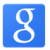## 如何从外部估算一个app的活跃用户数

为什么80%的码农都做不了架构师？->>>RT，主要关心日活跃用户数与月活跃用户数以及每日用户增长或流失情况。觉得是一个开放性的有意思的问题。大家有什么看法？

0a(0) = 1

a(n) = a(n-1) * K(0) + (20000 - a(n-1)) * K(1)

a为第N次装机的数量，K(0), K(1) 是常数系数，20000是饱和的用户装机量

b(0) = a(0) * N

b(n) = b(n-1) * N(0) + (a(n) - b(n-1)) * N(1)